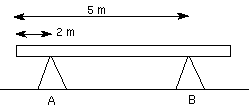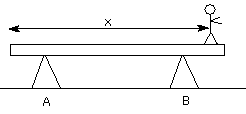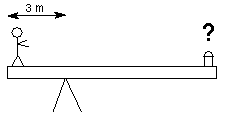# Painter Problem

davev

## Homework StatementA man of mass mm = 95 kg decides to paint his house. To do this, he builds a platform using a uniform beam with a mass of mb = 100 kg and a length of L = 7 meters. The beam is supported by two sawhorses, as shown in the diagram above.

a)
If the man stands over the support at point B, calculate the force exerted by the beam on the support at A.

F = 490 N

b)How far from the end of the beam (the end closest to support A) does the person have to stand to unbalance the beam?

x =

c)Later that day, after thinking about how cool rotational dynamics really is, the man decides to conduct an experiment. He removes one of the supports and places the other as shown in the diagram. Standing at the end of the board, he has his daughter place paint cans, each of mass mc = 2.9375 kg, on the opposite end. How many cans will the girl have to place on the board to provide the best balance? (You may neglect the actual length of the board that both the man and the cans occupy. Assume both are points at the ends of the board.) Also, take the axis of rotation about the sawhorse.

Number of cans =

## Homework Equations

For part b, we'd solve for x: (some length) * m = xM

I don't understand where to go for part c.

## The Attempt at a Solution

I said (some length) was 1.5 and tried to multiply by 95 and divide by 100, and I keep getting the wrong answer. I don't know where to go for parts b and c.

## Answers and Replies

voko
Hint for b: at the point farthest from the left where the balance is still possible, the A support is completely unloaded.

Hint for b & c: write down the equation for torques.

davev
Net Force = 0 ... Force of B – mg – Mg = 0
Net Torque = 0

(0)*sin(90) + mg*(some length)*sin(90) - Mg*(x)*sin(90) = 0
(some length)*m = xM

I just don't understand why 1.5 isn't the (some length) value for part b.

I don't understand part c still.

voko
I do not understand what "some length" is supposed to mean to begin with. Nor do I understand what "m" and "M" are. It would help if you stated what forces are present in case b, and where they are applied, so that their torques could be expressed unambiguously.

davev
m is 95 kg, and M is 100 kg. (some length) is a distance between A and B. (some length) + x = 3 m. I think that's correct, at least.

The two weights and a force pointing straight up are all that are present in part b.

voko
With respect to what you are computing the torques? Note it must be the same point for all the torques. What are the distances from that point?

davev
What do you mean? What is the distance from point B? Only two meters separate B and the end of the beam.

voko
What is unclear in my question "with respect to what you are computing the torques"?

davev
Maybe it's the wording, but I don't know what you're asking. I know that the setup I have for part b is right. That's how my professor set up his example in class. I just need to know what (some length), so I can find x.

Mentor
Net Force = 0 ... Force of B – mg – Mg = 0
Net Torque = 0

(0)*sin(90) + mg*(some length)*sin(90) - Mg*(x)*sin(90) = 0
(some length)*m = xM

I just don't understand why 1.5 isn't the (some length) value for part b.

I don't understand part c still.

Who says it's not?

voko
Maybe it's the wording, but I don't know what you're asking.

What is the definition of "torque"?